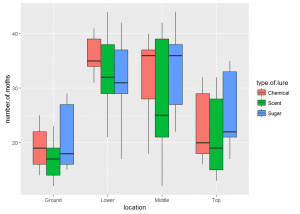Want to share your content on R-bloggers? click here if you have a blog, or here if you don't.One way analysis of variance helps us understand the relationship between one continuous dependent variable and one categorical independent variable. When we have one continuous dependent variable and more than one independent categorical variable we cannot use one way ANOVA. When we have two independent categorical variable we need to use two way ANOVA. When we have more than two categorical independent variables we need to use N way ANOVA.

In two way ANOVA there are three hypotheses of interest as listed below

1. H0: There is an effect of the first factor on the dependent continuous variable (main effect)
2. H0: There is an effect of the second factor variable on the dependent continuous variable (main effect)
3. H0: There is a combined effect of the first and second factor variable on the continuous dependent variable (interaction)

The above hypotheses can be extended from two factor variables to N factor variables.

For results of two way ANOVA to be valid there are several assumptions that need to be satisfied. They are listed below.

1. Observations must be independent within and across groups
2. Observations are approximately normally distributed.
3. There is equal variance in the observations
4. We should not have any outliers especially when our design is unbalanced
5. The errors are independent

When the normality and equal variance assumptions are violated you need to transform your data.

In this exercise we will use data on a moth experiment which is available here here. The data is not well formatted in that link so use this csv file moth-trap-experiment.

The dependent variable is the number of moths in a trap. The independent variables are location and type of lure. There were four locations (top, middle, lower and ground). There were three types of lure (scent, sugar and chemical).

Solutions to these exercises are found here

Exercise 1

Read in the data and inspect its structure

Exercise 2

Create summary statistics for location

Exercise 3

Create summary statistics for type of lure

Exercise 4

Create boxplots for each category

Exercise 5

Check for normality

Exercise 6

Check for equality of variance

Exercise 7

Take a log transformation of our data

Exercise 8

Perform a power analysis

Exercise 9

Perform anova

Exercise 10

Check homogeneity of variance